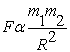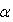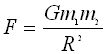#### The Law of Universal Gravitation

The force of attraction F between two objects with respective masses m1 and m2 separated by a center-to-center distance R, anywhere in the universe, is described by Newton's Law of Universal Gravitation:

The two postulates of this law are:

• The force of attraction is directly proportional to the product of the objects' masses

• The force of attraction is inversely proportional to the distance (taken from their respective centers) squared

If we combine the above statements mathematically, we obtain the following relation:This relationship is known as an inverse-square law.  This means that one factor (F) is inversely proportional to another factor (R) squared

We translate the above relationship into a definite equation by removing the "proportionalty symbol" () and replacing it with the "equal sign" (=) and a constant.  The symbol for this constant is "G"

Therefore:Where:

• F is the force of attraction between two masses m1 and m2 anywhere in the Universe, and
• and, G is the Universal Gravitational Constant: G = 6.67 X 10-11 Nm2 /Kg2The animation below is a very simple illustration of  the inverse-law lawNotes: When you use the above equation

• Observe proper units (mass in kg, distance in m, Force in [N]
• The distance R  has to be center-to center; i.e. from the center of one mass to the center of the other
• Don't forget to square the distance R
• Remember the significance of the Inverse Square Law